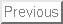Geometry

This chapter will deal with programming problems associated with real'' geometry - lines, points, circles, and so forth.

Although you did geometry in high school, it can be surprisingly difficult to program even very simple things. One reason is that floating point arithmetic introduces numerical uncertainty.

Another difficulty of geometric programming is that certain obvious'' operations you do with a pencil, such as finding the intersection of two lines, requires non-trivial programming to do correctly with a computer.

Finally, special cases or degeneracies require extra attention when doing geometric programming. For these reasons I recommend you carefully study my code fragments before writing your own.

There is more geometry to come next week, when we consider problems associated with line segments and polygons a field known as computational geometry,

Lines

Straight lines are the shortest distance between any two points. Lines are of infinite length in both directions, as opposed to line segments, which are finite. We limit our discussion here to lines in the plane.

Every line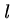is completely represented by any pair of pointsand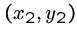which lie on it.

Lines are also completely described by equations such as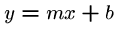, where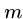is the slope of the line andis the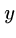-intercept, i.e., the unique point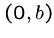where it crosses the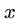-axis.

Vertical lines cannot be described by such equations, however, because dividing bymeans dividing by zero. The equation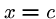denotes a vertical line that crosses the-axis at the point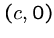.

We use the more general formula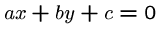as the foundation of our line type because it covers all possible lines in the plane:


typedef struct {
double a;               /* x-coefficient */
double b;               /* y-coefficient */
double c;               /* constant term */
} line;


Multiplying these coefficients by any non-zero constant yields an alternate representation for any line. We establish a canonical representation by insisting that the-coefficient equal 1 if it is non-zero. Otherwise, we set the-coefficient to 1:


points_to_line(point p1, point p2, line *l)
{
if (p1[X] == p2[X]) {
l->a = 1;
l->b = 0;
l->c = -p1[X];
} else {
l->b = 1;
l->a = -(p1[Y]-p2[Y])/(p1[X]-p2[X]);
l->c = -(l->a * p1[X]) - (l->b * p1[Y]);
}
}

point_and_slope_to_line(point p, double m, line *l)
{
l->a = -m;
l->b = 1;
l->c = -((l->a*p[X]) + (l->b*p[Y]));
}


Line Intersection

Two distinct lines have one intersection point unless they are parallel; in which case they have none. Parallel lines share the same slope but have different intercepts and by definition never cross.


bool parallelQ(line l1, line l2)
{
return ( (fabs(l1.a-l2.a) <= EPSILON) &&
(fabs(l1.b-l2.b) <= EPSILON) );
}


The intersection point of lines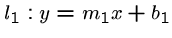and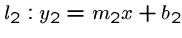is the point where they are equal, namely,intersection_point(line l1, line l2, point p)
{
if (same_lineQ(l1,l2)) {
printf("Warning: Identical lines, all points intersect.\n");
p[X] = p[Y] = 0.0;
return;
}

if (parallelQ(l1,l2) == TRUE) {
printf("Error: Distinct parallel lines do not intersect.\n");
return;
}

p[X] = (l2.b*l1.c - l1.b*l2.c) / (l2.a*l1.b - l1.a*l2.b);

if (fabs(l1.b) > EPSILON)       /* test for vertical line */
p[Y] = - (l1.a * (p[X]) + l1.c) / l1.b;
else
p[Y] = - (l2.a * (p[X]) + l2.c) / l2.b;
}


Angles

An angle is the union of two rays sharing a common endpoint.

The entire range of angles spans from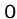to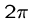radians or, equivalently,to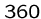degrees. Most trigonometric libraries assume angles are measured in radians.

A right angle measuresor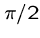radians.

Any two non-parallel lines intersect each other at a given angle. Lines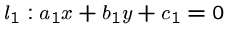and, written in the general form, intersect at the angle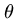given by: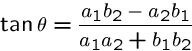For lines in slope-intercept form this reduces to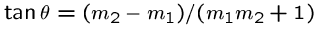.

Two lines are perpendicular if they cross at right angles to each other. The line perpendicular to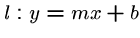is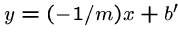, for all values of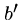.

Closest Point

A very useful subproblem is identifying the point on linewhich is closest to a given point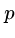. This closest point lies on the line throughwhich is perpendicular to, and hence can be found using the routines we have already developed:


closest_point(point p_in, line l, point p_c)
{
line perp;              /* perpendicular to l through (x,y) */

if (fabs(l.b) <= EPSILON) {     /* vertical line */
p_c[X] = -(l.c);
p_c[Y] = p_in[Y];
return;
}

if (fabs(l.a) <= EPSILON) {     /* horizontal line */
p_c[X] = p_in[X];
p_c[Y] = -(l.c);
return;
}

point_and_slope_to_line(p_in,1/l.a,&perp); /* normal case */
intersection_point(l,perp,p_c);
}


Triangles and Trigonometry

Each pair of rays with a common endpoint defines an internal angle of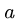radians and an external angle of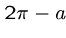radians. The three internal (smaller) angles of any triangle add up to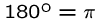radians.

The Pythagorean theorem enables us to calculate the length of the third side of any right triangle given the length of the other two. Specifically,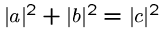, whereand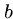are the two shorter sides, and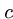is the longest side or hypotenuse.

We can go farther to analyze triangles using trigonometry. The trigonometric functions sine and cosine are defined as the- and-coordinates of points on the unit circle centered at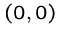. A third important trigonometric function is the tangent, defined as the ratio of sine over cosine.

These functions are important, because they enable us to relate the lengths of any two sides of a right triangle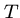with the non-right angles of. The non-hypotenuse edges can be labeled as opposite or adjacent edges in relation to a given angle. Then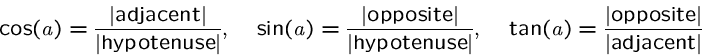Use the famous Indian Chief Soh-Cah-Toa to remember these relations, where each syllable of his name encodes a different relation. Cah'' means Cosine equals Adjacent over Hypotenuse, for example.

Using trigonometry described in the text, we can solve arbitrary triangles for lengths and angles given enough partial information.

Area of a Triangle

The areaof a triangleis given by, whereis the altitude andis the base of the triangle.

Another approach to computing the area of a triangle is directly from its coordinate representation. Using linear algebra and determinants, it can be shown that the signed area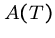of triangle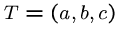is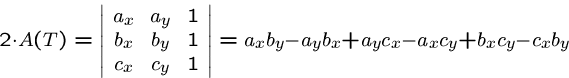This formula generalizes nicely to compute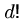times the volume of a simplex in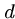dimensions.

Note that the signed areas can be negative, so we must take the absolute value to compute the actual area. The sign of this area can be used to build important primitives for computational geometry.


double signed_triangle_area(point a, point b, point c)
{
return( (a[X]*b[Y] - a[Y]*b[X] + a[Y]*c[X]
- a[X]*c[Y] + b[X]*c[Y] - c[X]*b[Y]) / 2.0 );
}

double triangle_area(point a, point b, point c)
{
return( fabs(signed_triangle_area(a,b,c)) );
}


Circles

A circle is defined as the set of points at a given distance (or radius) from its center,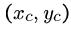. A circle can be represented in two basic ways, either as triples of boundary points or by its center/radius. For most applications, the center/radius representation is most convenient:


typedef struct {
point c;                /* center of circle */
double r;               /* radius of circle */
} circle;


The equation of a circle of radius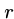follows directly from its center/radius representation,.

Many important quantities associated with circles are easy to compute. Specifically,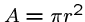and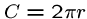.

A tangent lineintersects the boundary ofbut not its interior. The point of contact betweenandlies on the line perpendicular tothrough the center of. Since the triangle with side lengths,, andis a right triangle, we can compute the unknown tangent lengthusing the Pythagorean theorem. From, we can compute either the tangent point or the angle. The distancefromto the center is computed using the distance formula.

Two circles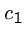and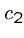of distinct radii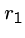and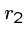will intersect if and only if the distance between their centers is at most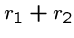.

The points of intersection form triangles with the two centers whose edge lengths are totally determined (,, and the distance between the centers), so the angles and coordinates can be computed as needed.

Assigned Problems

111301 (Dog and Gopher) - Find a hole for a slow gopher to hide from a fast dog. Is the closest hole really the safest spot for the gopher?

111302 (Rope Crisis in Ropeland!) - What is the length of the shortest rope between two points around a circular post

111305 (Birthday Cake) - How do you cut a circular cake such that both pieces are the same area and contain the same number of cherries? There is always a solution to this problem if we remove the constraint that the cutline have integer coordinates - can you prove it? Is there a more efficient solution than trying all possible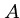,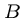pairs?

111308 (How Big Is It?) - What is the tightest way to order a non-overlapping collection of circles so as to minimize the `shadow' of them? Is it better to order the circles from largest to smallest, or to interleave them? Does the order ever not matter? Will backtracking work for this problem?